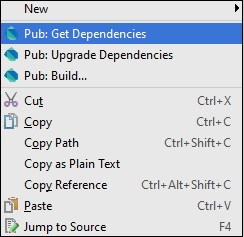# Dart Programming - Unit Testing

Unit Testing involves testing every individual unit of an application. It helps the developer to test small functionalities without running the entire complex application.

The Dart external library named "test" provides a standard way of writing and running unit tests.

Dart unit testing involves the following steps −

Step 1: Installing the "test" package

To installing third-party packages in the current project, you will require the pubspec.yaml file. To install test packages, first make the following entry in the pubspec.yaml file −

```dependencies:
test:
```

After making the entry, right-click the pubspec.yaml file and get dependencies. It will install the "test" package. Given below is a screenshot for the same in the WebStorm Editor.Packages can be installed from the command line too. Type the following in the terminal −

```pub get
```

Step 2: Importing the "test" package

```import "package:test/test.dart";
```

Step 3 Writing Tests

Tests are specified using the top-level function test(), while test assertions are made using the expect() function. For using these methods, they should be installed as a pub dependency.

### Syntax

```test("Description of the test ", () {
expect(actualValue , matchingValue)
});
```

The group() function can be used to group tests. Each group's description is added to the beginning of its test's descriptions.

### Syntax

```group("some_Group_Name", () {
test("test_name_1", () {
expect(actual, equals(exptected));
});
test("test_name_2", () {
expect(actual, equals(expected));
});
})
```

### Example 1: A Passing Test

The following example defines a method Add(). This method takes two integer values and returns an integer representing the sum. To test this add() method −

Step 1 − Import the test package as given below.

Step 2 − Define the test using the test() function. Here, the test() function uses the expect() function to enforce an assertion.

```import 'package:test/test.dart';
// Import the test package

// Function to be tested {
return x+y;
}
void main() {
// Define the test
// Arrange
var expected = 30;

// Act

// Asset
expect(actual,expected);
});
}
```

It should produce the following output

```00:00 +0: test to check add method
00:00 +1: All tests passed!
```

### Example 2: A Failing Test

The subtract() method defined below has a logical mistake. The following test verifies the same.

```import 'package:test/test.dart';
return x+y;
}
int Sub(int x,int y){
return x-y-1;
}
void main(){
test('test to check sub',(){
var expected = 10;
// Arrange

var actual = Sub(30,20);
// Act

expect(actual,expected);
// Assert
});
var expected = 30;
// Arrange

// Act

expect(actual,expected);
// Asset
});
}
```

Output − The test case for the function add() passes but the test for subtract() fails as shown below.

```00:00 +0: test to check sub
00:00 +0 -1: test to check sub
Expected: <10>
Actual: <9>
package:test  expect
bin\Test123.dart 18:5  main.<fn>

00:00 +0 -1: test to check add method
00:00 +1 -1: Some tests failed.
Unhandled exception:
Dummy exception to set exit code.
#0  _rootHandleUncaughtError.<anonymous closure> (dart:async/zone.dart:938)
#3  _Timer._runTimers (dart:isolate-patch/timer_impl.dart:394)
#4  _Timer._handleMessage (dart:isolate-patch/timer_impl.dart:414)
```

## Grouping Test Cases

You can group the test cases so that it adds more meaning to you test code. If you have many test cases this helps to write much cleaner code.

In the given code, we are writing a test case for the split() function and the trim function. Hence, we logically group these test cases and call it String.

### Example

```import "package:test/test.dart";
void main() {
group("String", () {
test("test on split() method of string class", () {
var string = "foo,bar,baz";
expect(string.split(","), equals(["foo", "bar", "baz"]));
});
test("test on trim() method of string class", () {
var string = "  foo ";
expect(string.trim(), equals("foo"));
});
});
}
```

Output − The output will append the group name for each test case as given below −

```00:00 +0: String test on split() method of string class
00:00 +1: String test on trim() method of string class
00:00 +2: All tests passed
```